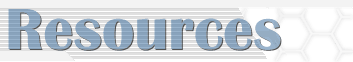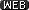Math Portal Home > Performance Tasks

Performance Tasks for Algebraic Thinking for K- 5

This section contains a number of questions for students to answer. The tasks also contain the specific Sunshine State Standards being used. These tasks were originally developed as part of a DOE supported project, Algebraic Thinking for K-5, directed by Dr. Michael Hynes at the University of Central Florida.IntroductionLizards and Snakes: working with logicPocket Count: analyzing graphsQuilting Pattern: determining patterns on a quiltSeating Problem: arranging seats at a table according to a patternSeal Problem: deciding how much a cube and a cone weighStairs: building sets of stairs, creating patterns with stairs

In this section, objectives are provided to achieve a mathematical goal. Each lesson plan is correlated to the Sunshine State Standards and included a list of materials needed, recommendations for student arrangement, and performance criteria. These tasks were originally developed by Pinellas County teachers.Elementary Mathematics Performance Tasks Overview: what are performance tasks and how can they be scored?Grade 5 Performance Tasks Answer Key: answers to all performance tasksAdding and Subtracting Decimals: constructing a bar graph, analyzing dataAdding and Subtracting Fractions: adding and subtracting fractions with like and unlike denominatorsDividing Whole Numbers: 1-Digit Divisors: dividing by 1-digit numbers, finding mean, median and modeDividing Whole Numbers: 2-Digit Divisors: dividing by 2-digit divisors, solving to find the least and greatest quotientGeometry: understanding intersecting, parallel and perpendicular lines, identifying angles, symmetry and congruencyRatio and Probability: finding the probability of an eventMultiplying and Dividing Decimals: multiplying whole numbers by decimals, dividing decimals by whole numbersMultiplying and Dividing Fractions: multiplying whole numbers and fractions, working with range of numbersMultiplying Whole Numbers: multiplying up to 5-digit numbers by 1-digit numbers, finding areaUnderstanding Fractions and Mixed Numbers: reading and writing fractions and mixed numbers, estimating lengthsUnderstanding Numbers: reading and writing whole numbers to billions, comparing whole numbersUsing Addition and Subtraction: estimating and calculating sums and differences of 3-digit to 6-digit numbersCorn Crop: planning a garden working with shapes and positioning of plantsHow Big Are We?: measuring height and lengthsTrail Mix: reading a letter and determining if the fractions are correctSnack Mix: making a snack for a group, working with fractions
These resources can be viewed in two ways. To view a web version of the resources click on thebuttons. To view the resources in a printable version click on thebuttons. The Adobe Acrobat Reader plug-in is required to view these documents. Go to the Adobe site to download this free plug-in.